### Home > PC > Chapter 4 > Lesson 4.3.1 > Problem4-130

4-130.
1.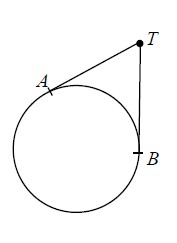A string around a circle is pulled up by a thumbtack, T, as shown below. The string is tangent to the circle with AT = TB. Given that the circle has a radius of r, complete the following problems. Homework Help ✎

1. Find an expression for the length of the string if AT = r.

2. How long would the string have to be if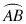is 120°?

3. Find mATB.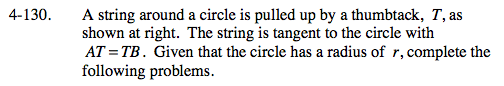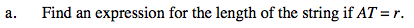1.5πr + 2r ≈ 6.712r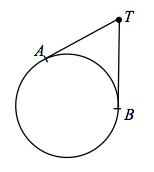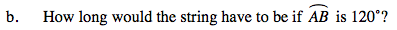$\frac{4\pi r}{3}+2r\sqrt3\approx 7.653\textit{r}$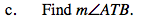60°